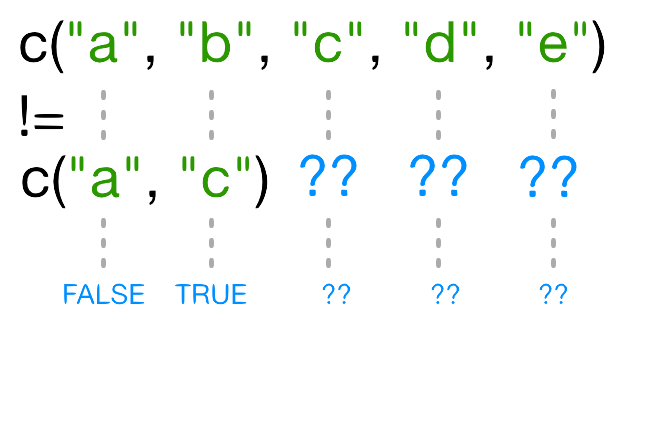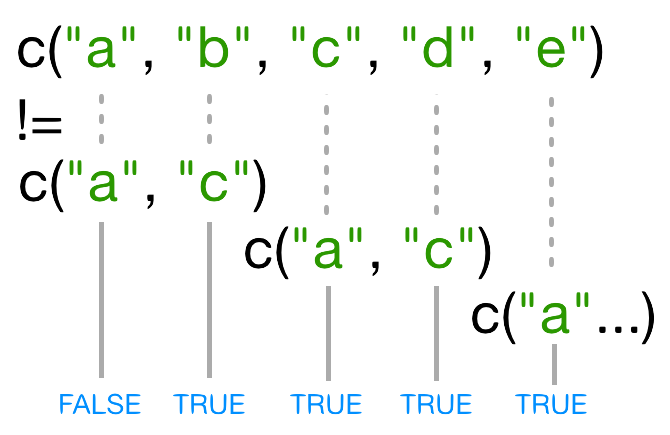# Subsetting data and vectorization part I

## Instructions:

Use the left and right arrow keys to navigate the presentation forward and backward respectively. You can also use the arrows at the bottom right of the screen to navigate with a mouse.

FAIR USE ACT DISCLAIMER:
This site is for educational purposes only. This website may contain copyrighted material, the use of which has not been specifically authorized by the copyright holders. The material is made available on this website as a way to advance teaching, and copyright-protected materials are used to the extent necessary to make this class function in a distance learning environment. The Fair Use Copyright Disclaimer is under section 107 of the Copyright Act of 1976, allowance is made for “fair use” for purposes such as criticism, comment, news reporting, teaching, scholarship, education and research.

## Outline

• The following topics will be covered in this lecture:

• More on subsetting data
• Skipping and removing elements
• Subsetting based on logical values and other conditions

## More on subsetting data

• R has many powerful subset operators – mastering them will allow you to easily perform complex operations on any kind of dataset.

• There are six different ways we can subset any kind of object, and three different subsetting operators for the different data structures.

• Let's start with the workhorse of R: a simple numeric vector.

``````x <- c(5.4, 6.2, 7.1, 4.8, 7.5)
names(x) <- c('a', 'b', 'c', 'd', 'e')
x
``````
``````  a   b   c   d   e
5.4 6.2 7.1 4.8 7.5
``````
• In R, simple vectors containing character strings, numbers, or logical values are called atomic vectors because they can't be further simplified.

## Accessing elements using their indices

• So now that we've created a dummy vector to play with, how do we get at its contents?

• To extract elements of a vector we can give their corresponding index, starting from one:

``````x
``````
``````  a
5.4
``````
``````x
``````
``````  d
4.8
``````
• It may look different, but the square brackets operator is a function.

• For vectors (and matrices), it means “get me the nth element”.

### Accessing elements using their indices – continued

• We can ask for multiple elements at once:
``````x[c(1, 3)]
``````
``````  a   c
5.4 7.1
``````
• Or slices of the vector:
``````x[1:4]
``````
``````  a   b   c   d
5.4 6.2 7.1 4.8
``````
• The `:` operator creates a sequence of numbers from the left element to the right.
``````1:4
``````
`````` 1 2 3 4
``````
``````c(1, 2, 3, 4)
``````
`````` 1 2 3 4
``````

### Accessing elements using their indices – continued

• We can ask for the same element multiple times:
``````x[c(1,1,3)]
``````
``````  a   a   c
5.4 5.4 7.1
``````
• If we ask for an index beyond the length of the vector, R will return a missing value:
``````x
``````
``````<NA>
NA
``````
• This is a vector of length one containing an `NA`, whose name is also `NA`.

### Accessing elements using their indices – continued

• If we ask for the 0th element, we get an empty vector:
``````x
``````
``````named numeric(0)
``````
• In many programming languages (C and Python, for example), the first element of a vector has an index of 0.

• In R, the first element is 1.

## Skipping and removing elements

• If we use a negative number as the index of a vector, R will return every element except for the one specified:
``````x[-2]
``````
``````  a   c   d   e
5.4 7.1 4.8 7.5
``````
• We can skip multiple elements:
``````x[c(-1, -5)]  # or x[-c(1,5)]
``````
``````  b   c   d
6.2 7.1 4.8
``````
• A common trip up for novices occurs when trying to skip slices of a vector.

• It's natural to try to negate a slice as follows

``````x[-1:3]
``````
• But this gives a somewhat cryptic error…
``````Error in x[-1:3]: only 0's may be mixed with negative subscripts
``````

### Skipping and removing elements – continued

• The key to understanding this is remembering the order of operations.

• `:` is really a function, which we want to respect the arguments of.
• It takes its first argument as -1, and its second as 3, so generates the sequence of numbers: `c(-1, 0, 1, 2, 3)`.

• The correct solution is to wrap that function call in brackets, so that the `-` operator applies to the result:

``````x[-(1:3)]
``````
``````  d   e
4.8 7.5
``````
• To remove elements from a vector, we need to assign the result back into the variable:
``````x <- x[-4]
x
``````
``````  a   b   c   e
5.4 6.2 7.1 7.5
``````

## Subsetting by name

• We can extract elements by using their name, instead of extracting by index:
``````x <- c(a=5.4, b=6.2, c=7.1, d=4.8, e=7.5) # we can name a vector 'on the fly'
x[c("a", "c")]
``````
``````  a   c
5.4 7.1
``````
• This is usually a much more reliable way to subset objects:

• the position of various elements can often change when chaining together subsetting operations, but the names will always remain the same.

## Subsetting through other logical operations

• We can also use any logical vector to subset:
``````x[c(FALSE, FALSE, TRUE, FALSE, TRUE)]
``````
``````  c   e
7.1 7.5
``````
• Since comparison operators (e.g. `>`, `<`, `==`) evaluate to logical vectors, we can also use them to succinctly subset vectors:

• the following statement gives the same result as the previous one.
``````x[x > 7]
``````
``````  c   e
7.1 7.5
``````

## Subsetting through other logical operations

• Breaking it down, this statement first evaluates `x>7`,

• generating a logical vector `c(FALSE, FALSE, TRUE, FALSE, TRUE)`,
• and then selects the elements of `x` corresponding to the `TRUE` values.
• We can use `==` to mimic the previous method of indexing by name (remember you have to use `==` rather than `=` for comparisons):

``````x[names(x) == "a"]
``````
``````  a
5.4
``````

### Combining logical conditions

• We often want to combine multiple logical criteria.

• For example, we might want to find all the countries that are located in Asia or Europe and have life expectancies within a certain range.

• Several operations for combining logical vectors exist in R:

• `&`, the “logical AND” operator: returns `TRUE` if both the left and right are `TRUE`.
• `|`, the “logical OR” operator: returns `TRUE`, if either the left or right (or both) are `TRUE`.

### Combining logical conditions

• You may sometimes see `&&` and `||` instead of `&` and `|`.

• These two-character operators only look at the first element of each vector and ignore the remaining elements. In general you should not use the two-character operators in data analysis;

• save them for programming, i.e. deciding whether to execute a statement.

• `!`, the “logical NOT” operator: converts `TRUE` to `FALSE` and `FALSE` to `TRUE`.

• It can negate a single logical condition (eg `!TRUE` becomes `FALSE`), or a whole vector of conditions(eg `!c(TRUE, FALSE)` becomes `c(FALSE, TRUE)`).

• Additionally, you can compare the elements within a single vector using the `all` function (which returns `TRUE` if every element of the vector is `TRUE`) and the `any` function (which returns `TRUE` if one or more elements of the vector are `TRUE`).

### Non-unique names

• You should be aware that it is possible for multiple elements in a vector to have the same name. (For a data frame, columns can have the same name;

• Consider this example:

`````` x <- 1:3
x
``````
`````` 1 2 3
``````
``````names(x) <- c('a', 'a', 'a')
x
``````
``````a a a
1 2 3
``````

### Non-unique names

• Now consider if we try to extract data by name when all elements have the same name:
``````x['a']  # only returns first value
``````
``````a
1
``````
``````x[names(x) == 'a']  # returns all three values
``````
``````a a a
1 2 3
``````

## Skipping named elements

• Skipping or removing named elements is a little harder. If we try to skip one named element by negating the string, R complains (slightly obscurely) that it doesn't know how to take the negative of a string:
``````x <- c(a=5.4, b=6.2, c=7.1, d=4.8, e=7.5) # we start again by naming a vector 'on the fly'
x[-"a"]
``````
``````Error in -"a": invalid argument to unary operator
``````
• However, we can use the `!=` (not-equals) operator to construct a logical vector that will do what we want:
``````x[names(x) != "a"]
``````
``````  b   c   d   e
6.2 7.1 4.8 7.5
``````

## Skipping named elements

• Skipping multiple named indices is a little bit harder still. Suppose we want to drop the `"a"` and `"c"` elements, so we try this:
``````x[names(x)!=c("a","c")]
``````
``````  b   c   d   e
6.2 7.1 4.8 7.5
``````
• R did something, but it gave us a warning that we ought to pay attention to - and it apparently gave us the wrong answer (the `"c"` element is still included in the vector)!

• So what does `!=` actually do in this case? That's an excellent question…

### Recycling

• Let's take a look at the comparison component of this code:
``````names(x) != c("a", "c")
``````
`````` FALSE  TRUE  TRUE  TRUE  TRUE
``````
• When you use `!=`, R tries to compare each element of the left argument with the corresponding element of its right argument.

• What happens when you compare vectors of different lengths?

### Recycling – continued• When one vector is shorter than the other, it gets recycled• In this case R repeats c(“a”, “c”) as many times as necessary to match names(x), i.e. we get c(“a”,“c”,“a”,“c”,“a”).
• Since the recycled “a” doesn’t match the third element of names(x), the value of != is TRUE.
• Because in this case the longer vector length (5) isn’t a multiple of the shorter vector length (2), R printed a warning message.
• If we had been unlucky and names(x) had contained six elements, R would silently have done the wrong thing (i.e., not what we intended it to do).
• This recycling rule can can introduce hard-to-find and subtle bugs.

### Recycling

• The way to get R to do what we really want (match each element of the left argument with all of the elements of the right argument) it to use the `%in%` operator.

• The `%in%` operator goes through each element of its left argument, in this case the names of `x`, and asks, “Does this element occur in the second argument?”.

• Here, since we want to exclude values, we also need a `!` operator to change “in” to “not in”:

``````x[! names(x) %in% c("a","c") ]
``````
``````  b   d   e
6.2 4.8 7.5
``````

## Handling special values

• At some point you will encounter functions in R that cannot handle missing, infinite, or undefined data.

• There are a number of special functions you can use to filter out this data:

• `is.na` will return all positions in a vector, matrix, or data.frame containing `NA` (or `NaN`)
• likewise, `is.nan`, and `is.infinite` will do the same for `NaN` and `Inf`.
• `is.finite` will return all positions in a vector, matrix, or data.frame that do not contain `NA`, `NaN` or `Inf`.
• `na.omit` will filter out all missing values from a vector